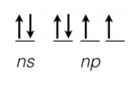# Problem: The partial orbital diagram below (where n could be any valid quantum number) describes the valence electrons for which of the atoms below? (a) Nitrogen (b) Silicon (c) Bromine (d) Sulfur (e) Gallium

###### FREE Expert Solution
91% (255 ratings)
###### Problem Details

The partial orbital diagram below (where n could be any valid quantum number) describes the valence electrons for which of the atoms below?

(a) Nitrogen

(b) Silicon

(c) Bromine

(d) Sulfur

(e) GalliumWhat scientific concept do you need to know in order to solve this problem?

Our tutors have indicated that to solve this problem you will need to apply the Orbital Diagrams concept. If you need more Orbital Diagrams practice, you can also practice Orbital Diagrams practice problems.

What is the difficulty of this problem?

Our tutors rated the difficulty ofThe partial orbital diagram below (where n could be any vali...as medium difficulty.

How long does this problem take to solve?

Our expert Chemistry tutor, Jules took undefined 40 seconds to solve this problem. You can follow their steps in the video explanation above.

What professor is this problem relevant for?

Based on our data, we think this problem is relevant for Professor Arman's class at UTSA.